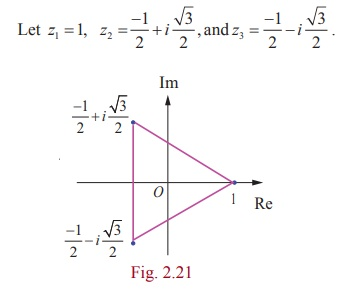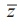Home | | Maths 12th Std | Modulus of a Complex Number: Solved Example Problems

# Modulus of a Complex Number: Solved Example Problems

Mathematics : Complex Numbers: Modulus of a Complex Number: Solved Example Problems with Answers, Solution

### Example 2.9

If z1 = 3 + 4i, z2 = 5 -12i, and z3 = 6 + 8i, find |z1| , |z2|, |z3|, |z1 + z2| ,| z2 - z3|, and |z1 + z3|.

### Solution

Using the given values for z1, z2 and z3 we get |z1| = |3 + 4i| = ŌłÜ[32 +42] =5

|z2|   = |5 -12i| = ŌłÜ[52 + (-12)2] = 13

z3   = |6 + 8i| = ŌłÜ[62 + 82] = 10

|z1  + z2| =  |(3 + 4i ) + (5 -12i)| = |8 - 8i| = ŌłÜ128 = 8ŌłÜ2

|z2 - z3| = |(5 -12i ) - (6 + 8i )| = |-1- 20i| = ŌłÜ401

|z1  + z3| =  |(3 + 4i) + (6 + 8i)| = |9 +12i| = ŌłÜ225 = 15

Note that the triangle inequality is satisfied in all the cases.

|z1 + z3| = |z1| + |z3|= 15 (why?)

Example 2.10

Find the followingSolutionExample 2.11

Which one of the points i, -2 + i , and 3 is farthest from the origin?

Solution

The distance between origin to z = i, -2 + i, and 3 are

| z | = | i | = 1

| z | = | -2 + i |= ŌłÜ[(-2)2+(1)2] = ŌłÜ5

= | z | = | 3 | = 3Since 1 < ŌłÜ5 < 3 , the farthest point from the origin is 3

Example 2.12

If z1, z2, and z3 are complex numbers such that |z1| = |z2| =|z3| = |z1 + z2 + z3| = 1, find the value of.

Solution

Since, |z1| = |z1| = |z1| = 1,Example 2.13

If |z| = 2 show that 3 Ōēż |z + 3 + 4i| Ōēż 7

Solution

|z + 3 + 4i| Ōēż |z| + |3 + 4i|  = 2 + 5 = 7

|z + 3 + 4i| Ōēż 7      (1)|z + 3 + 4i| Ōēź | |z| - | 3 + 4i| | = |2-5| = 3

|z + 3 + 4i| Ōēź 3                     (2)

From (1) and (2), we get 3 Ōēż |z + 3 + 4i| Ōēż 7

Note

To find the lower bound and upper bound use | |z1| - |z2| | Ōēż |z1 + z2 | Ōēż |z1| + |z2|.

Example 2.14

Show that the points 1,are the vertices of an equilateral triangle.

Solution

It is enough to prove that the sides of the triangle are equal.

Let z = 1,The length of the sides of the triangles areSince the sides are equal, the given points form an equilateral triangle.

Example 2.15

Let z1 , z2 , and z3 be complex numbers such that |z1| = |z2| =|z3| = r > 0 and z1 + z2 + z3 ŌēĀ 0 .

Prove that.

SolutionExample 2.16

Show that the equation z2has four solutions.

Solution

We have,It has 3 non-zero solutions. Hence including zero solution, there are four solutions.

Tags : with Answers, Solution , 12th Mathematics : UNIT 2 : Complex Numbers
Study Material, Lecturing Notes, Assignment, Reference, Wiki description explanation, brief detail
12th Mathematics : UNIT 2 : Complex Numbers : Modulus of a Complex Number: Solved Example Problems | with Answers, Solution